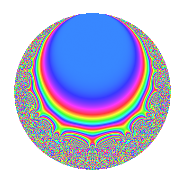# Properties

 Label 27.8.cLevel 27 Weight 8 Character orbit c Rep. character $$\chi_{27}(10,\cdot)$$ Character field $$\Q(\zeta_{3})$$ Dimension 12 Newforms 1 Sturm bound 24 Trace bound 0

# Related objects

## Defining parameters

 Level: $$N$$ = $$27 = 3^{3}$$ Weight: $$k$$ = $$8$$ Character orbit: $$[\chi]$$ = 27.c (of order $$3$$ and degree $$2$$) Character conductor: $$\operatorname{cond}(\chi)$$ = $$9$$ Character field: $$\Q(\zeta_{3})$$ Newforms: $$1$$ Sturm bound: $$24$$ Trace bound: $$0$$

## Dimensions

The following table gives the dimensions of various subspaces of $$M_{8}(27, [\chi])$$.

Total New Old
Modular forms 48 16 32
Cusp forms 36 12 24
Eisenstein series 12 4 8

## Trace form

 $$12q + 9q^{2} - 321q^{4} + 180q^{5} - 84q^{7} - 5922q^{8} + O(q^{10})$$ $$12q + 9q^{2} - 321q^{4} + 180q^{5} - 84q^{7} - 5922q^{8} + 252q^{10} + 8460q^{11} - 1848q^{13} + 16272q^{14} - 12417q^{16} - 30564q^{17} + 24432q^{19} + 40788q^{20} - 35001q^{22} + 51588q^{23} + 4746q^{25} - 536472q^{26} + 75516q^{28} + 414648q^{29} + 8196q^{31} + 1048977q^{32} - 106623q^{34} - 2210616q^{35} + 139344q^{37} + 1952685q^{38} + 305496q^{40} + 1731582q^{41} + 408372q^{43} - 5169114q^{44} - 1684008q^{46} + 1631484q^{47} - 179010q^{49} + 1654461q^{50} + 681594q^{52} - 2835648q^{53} - 16056q^{55} + 1784466q^{56} - 948384q^{58} + 2055636q^{59} - 2723196q^{61} + 1026828q^{62} + 7178178q^{64} + 1387620q^{65} + 3806556q^{67} - 2142639q^{68} + 953442q^{70} - 2408400q^{71} - 10670052q^{73} - 9846504q^{74} - 6727827q^{76} - 3478824q^{77} + 6020916q^{79} + 38072448q^{80} + 9403002q^{82} - 9605052q^{83} - 1698624q^{85} - 34278561q^{86} - 16459029q^{88} + 24630264q^{89} + 13570104q^{91} - 39143394q^{92} + 12602808q^{94} - 10422072q^{95} + 9977226q^{97} + 95833314q^{98} + O(q^{100})$$

## Decomposition of $$S_{8}^{\mathrm{new}}(27, [\chi])$$ into irreducible Hecke orbits

Label Dim. $$A$$ Field CM Traces $q$-expansion
$$a_2$$ $$a_3$$ $$a_5$$ $$a_7$$
27.8.c.a $$12$$ $$8.434$$ $$\mathbb{Q}[x]/(x^{12} - \cdots)$$ None $$9$$ $$0$$ $$180$$ $$-84$$ $$q+(-\beta _{1}+\beta _{6}+\beta _{7})q^{2}+(-52+3\beta _{6}+\cdots)q^{4}+\cdots$$

## Decomposition of $$S_{8}^{\mathrm{old}}(27, [\chi])$$ into lower level spaces

$$S_{8}^{\mathrm{old}}(27, [\chi]) \cong$$ $$S_{8}^{\mathrm{new}}(9, [\chi])$$$$^{\oplus 2}$$